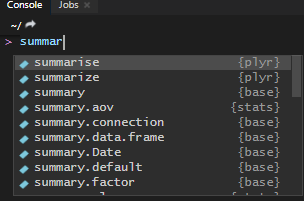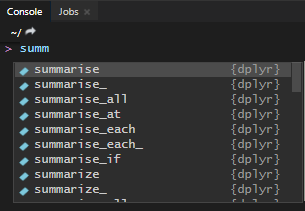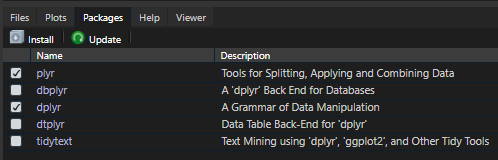# dplyr error: `n()` must only be used inside dplyr verbs

If you are using R and getting dplyr error: `n()` must only be used inside dplyr verbs, then here is the most likely cause and 3 possible solutions to this problem.

## The cause of the error: `n()` must only be used inside dplyr verbs

The cause of the error: `n()` must only be used inside dplyr verbs usually is simple – multiple packages are using a function with the same name. If you are using a function `n()` inside dplyr functions `summarise()` or `summarize()`, then look at what kind of package they are referring to.

Usually, the cause of this problem is that plyr or Hmisc packages are loaded.This situation is not unique, and it might happen that you are not using the dplyr version of the function. Here is another one – dplyr error in select: unused argument.

The same problem might happen with the `count` function in dplyr that is also in plyr.
The dplyr `count` function is very useful, and here is more about that in my top 10 favorite dplyr tips and tricks.

## Solutions to error: must only be used inside dplyr verbs

2. use R namespace
3. detach R package

If you prefer working with function from the dplyr, then there is a simple solution – load dplyr package last.

In this scenario, `summarize` will refer to the dplyr version of this function.

```require(plyr)
require(dplyr)```If I do this in the opposite order, the function referring to plyr.

```require(dplyr)
require(plyr)```## 2. use R namespace

Use dplyr package namespace for functions to prevent unintentional use with other packages like plyr in this case.
Here is how it looks with the `summarise` function.

```require(dplyr)
require(plyr)

iris %>% filter(Species == 'setosa') %>% dplyr::summarise(n())```

Here is a great resource if you want to read more about R namespaces.

If you’re using custom functions, then it might be useful to assign it to some package namespace. Here is how to do that with the function that copies data from R to Excel.

## 3. detach R package

Detach plyr package if it is no longer needed. You can unload dplyr or any other R package without restarting R or RStudio by using detach function.

`detach("package:plyr", unload = TRUE)`

If you forget about this function or how to use that, try to use checkboxes in the RStudio packages tab.Posted

in